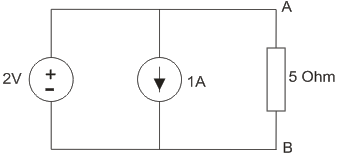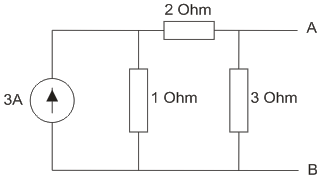# MCQs on Electric Circuits

##### Page 50 of 63. Go to page 1 2 3 4 5 6 7 8 9 10 11 12 13 14 15 16 17 18 19 20 21 22 23 24 25 26 27 28 29 30 31 32 33 34 35 36 37 38 39 40 41 42 43 44 45 46 47 48 49 50 51 52 53 54 55 56 57 58 59 60 61 62 63
01․ Which of the following ABCD parameters is unit less?
A and D.
A and B.
B and C.
A and C.

A = V1/V2 and D = I1/I2. They are ratios of voltages and currents respectively.

02․ Condition of reciprocity in Y-parameter representation is
Y11 = Y12.
Y12 = Y21.
Y11 = Y22.
Y12 = Y22.

A two-port network is said to be reciprocal if the ratio of the excitation to the response is invariant to an interchange of the positions of the excitation and response in the network. This will hold good, if Y12 = Y21.

03․ Cascade connection of two port networks is analyzed through
ABCD parameters.
Z parameters.
Y parameters.
h parameters.

In cascade connection, the output of one network acts as the input of the next network. ABCD parameters relate the input parameters of a two port network in terms of the output parameters. Hence, the cascade connection is analyzed through ABCD parameters.

04․ Condition of symmetry in Z-parameter representation is
Z11 = Z12.
Z11 = Z22.
Z12 = Z21.
Z12 = Z22.

A two-port network is said to be symmetrical if the ports of the two-port network can be interchanged without changing the port voltages and currents. This will hold good, if Z11 = Z22.

05․ Condition of symmetry in ABCD parameter representation is
A = D.
C = D.
B = D.

A two-port network is said to be symmetrical if the ports of the two-port network can be interchanged without changing the port voltages and currents. This will hold good, if A = D.

06․ Find the current through 5 Ohm resistor from A to B.0.4A
1.4A.
-0.4A.
-1.4A.

Applying Superposition theorem, current due to 2V source is 0.4A from A to B; current due to 1A source is zero from B to A as all current will flow through the shorted 2V source. So total current from A to B due to all the sources is 0.4A.

07․ Which of the following is applicable for both linear and non-linear circuits?
None of these .
Thevnin’s theorem.
Norton’s theorem.
Superposition theorem.

All these theorems are applicable for circuits that are linear.

08․ While Thevenizing a circuit between two terminals, VTH is equal to
open circuit terminal voltage .
short circuit terminal voltage.
net voltage available in the circuit.
none of these.

The thevnin voltage is given by the open circuit voltage across the terminals.

09․ The thevnin equivalent of the circuit shown in the figure is0.75V, 5/6 Ohm
0.75 V, 1.5 Ohm
1.5V, 1.5 Ohm
1.5V, 5/6 Ohm

To find the thevnin resistance, the current source is open circuited and the equivalent resistance looking into the circuit from AB terminals is 3|| (2+1) = 1.5 Ohm. To find the thevnin voltage, the current through the 3 Ohm resistance is 3×1/(1+2+3) = 0.5A; So open source voltage across the terminals is 3×0.5 = 1.5V

10․ The norton equivalent of the circuit shown in the figure is0.75A, 5/6 Ohm
0.75 A, 1.5 Ohm
1A, 1.5 Ohm
1A, 5/6 Ohm

To find the norton resistance, the current source is open circuited and the equivalent resistance looking into the circuit from AB terminals is 3|| (2+1) = 1.5 Ohm. To find the Norton current, the terminals AB re short circuited and the current bypasses the 3 Ohm resistance. So the current through the short circuited AB terminals is 3×1/(1+2) = 1A.

<<<4849505152>>>